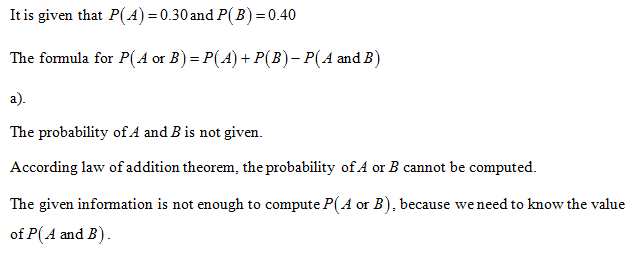# You are given the information that P(A) = 0.30 and P(B) = 0.40.(a) Do you have enough information to compute P(A or B)? Explain.No. You need to know the value of P(A) + P(B).No. You need to know the value of P(A and B).    Yes. This probability is equal to 0.70.No. You need to know the value of P(A) - P(B).(b) If you know that events A and B are mutually exclusive, do you have enough information to compute P(A or B)? Explain.Yes. P(A and B) = 0.12, so P(A or B) = P(A) + P(B) - 0.12.Yes. P(A and B) = 0, so P(A or B) = P(B) - P(A).    No. Knowing the events are mutually exclusive does not provide any extra information.Yes. P(A and B) = 0, so P(A or B) = P(A) + P(B).

Question
15 views
You are given the information that P(A) = 0.30 and P(B) = 0.40.
(a) Do you have enough information to compute P(A or B)? Explain.
No. You need to know the value of P(A) + P(B).No. You need to know the value of P(A and B).    Yes. This probability is equal to 0.70.No. You need to know the value of P(A) - P(B).

(b) If you know that events A and B are mutually exclusive, do you have enough information to compute P(A or B)? Explain.
Yes. P(A and B) = 0.12, so P(A or B) = P(A) + P(B) - 0.12.Yes. P(A and B) = 0, so P(A or B) = P(B) - P(A).    No. Knowing the events are mutually exclusive does not provide any extra information.Yes. P(A and B) = 0, so P(A or B) = P(A) + P(B).

check_circle

Step 1...

### Want to see the full answer?

See Solution

#### Want to see this answer and more?

Solutions are written by subject experts who are available 24/7. Questions are typically answered within 1 hour.*

See Solution
*Response times may vary by subject and question.
Tagged in